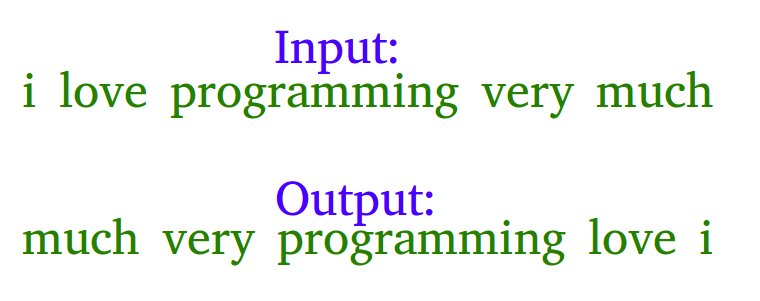# C Program To Reverse Words In A Given String

• Last Updated : 15 Dec, 2021

Example: Let the input string be “i like this program very much”. The function should change the string to “much very program this like i”Examples

Input: s = “geeks quiz practice code”
Output: s = “code practice quiz geeks”

Input: s = “getting good at coding needs a lot of practice”
Output: s = “practice of lot a needs coding at good getting”

Algorithm:

• Initially, reverse the individual words of the given string one by one, for the above example, after reversing individual words the string should be “i ekil siht margorp yrev hcum”.
• Reverse the whole string from start to end to get the desired output “much very program this like i” in the above example.

Below is the implementation of the above approach:

## C

 `// C program to reverse a string ` `#include ` ` `  `// Function to reverse any sequence ` `// starting with pointer begin and ` `// ending with pointer end ` `void` `reverse(``char``* begin,  ` `             ``char``* end) ` `{ ` `    ``char` `temp; ` `    ``while` `(begin < end)  ` `    ``{ ` `        ``temp = *begin; ` `        ``*begin++ = *end; ` `        ``*end-- = temp; ` `    ``} ` `} ` ` `  `// Function to reverse words ` `void` `reverseWords(``char``* s) ` `{ ` `    ``char``* word_begin = s; ` ` `  `    ``// Word boundary ` `    ``char``* temp = s; ` ` `  `    ``// Reversing individual words as ` `    ``// explained in the first step ` `    ``while` `(*temp)  ` `    ``{ ` `        ``temp++; ` `        ``if` `(*temp == ``''``)  ` `        ``{ ` `            ``reverse(word_begin,  ` `                    ``temp - 1); ` `        ``} ` `        ``else` `if` `(*temp == ``' '``)  ` `        ``{ ` `            ``reverse(word_begin,  ` `                    ``temp - 1); ` `            ``word_begin = temp + 1; ` `        ``} ` `    ``} ` ` `  `    ``// Reverse the entire string ` `    ``reverse(s, temp - 1); ` `} ` ` `  `// Driver Code ` `int` `main() ` `{ ` `    ``char` `s[] =  ` `    ``"i like this program very much"``; ` `    ``char``* temp = s; ` `    ``reverseWords(s); ` `    ``printf``(``"%s"``, s); ` `    ``return` `0; ` `} `

Output:

`much very program this like i`

The above code doesn’t handle the cases when the string starts with space. The following version handles this specific case and doesn’t make unnecessary calls to reverse function in the case of multiple spaces in between. Thanks to rka143 for providing this version.

## C

 `// C program to implement ` `// the above approach ` `void` `reverseWords(``char``* s) ` `{ ` `    ``char``* word_begin = NULL; ` `   `  `    ``// temp is for word boundary  ` `    ``char``* temp = s; ` ` `  `    ``// STEP 1 of the above algorithm ` `    ``while` `(*temp)  ` `    ``{ ` `        ``/*This condition is to make sure  ` `          ``that the string start with valid  ` `          ``character (not space) only*/` `        ``if` `((word_begin == NULL) &&  ` `            ``(*temp != ``' '``))  ` `        ``{ ` `            ``word_begin = temp; ` `        ``} ` `        ``if` `(word_begin &&  ` `           ``((*(temp + 1) == ``' '``) || ` `            ``(*(temp + 1) == ``''``)))  ` `        ``{ ` `            ``reverse(word_begin, temp); ` `            ``word_begin = NULL; ` `        ``} ` `        ``temp++; ` `    ``// End of while  ` `    ``}  ` ` `  `    ``// STEP 2 of the above algorithm  ` `    ``reverse(s, temp - 1); ` `} `

Time Complexity: O(n)
Another Approach:

Please refer complete article on Reverse words in a given string for more details!

My Personal Notes arrow_drop_up
Recommended Articles
Page :